# Electrostatic Analysis of Transformer Bushing Insulator

This example shows how to compute the electric field intensity in a bushing insulator of a transformer. Bushing insulators must withstand large electric fields due to the potential difference between the ground and the high-voltage conductor. This example uses a 3-D electrostatic model to compute the voltage distribution and electric field intensity in the bushing.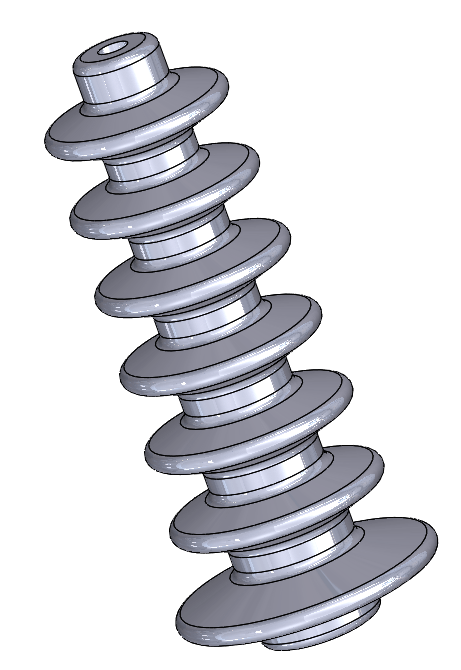Create an electromagnetic model for electrostatic analysis.

`model = createpde('electromagnetic','electrostatic');`

Import and plot the bushing geometry.

```gmBushing = importGeometry('TransformerBushing.stl'); pdegplot(gmBushing)```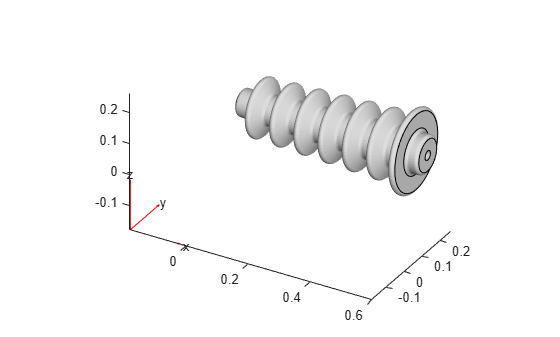Model the surrounding air as a cuboid, and position the cuboid to contain the bushing at its center.

```gmAir = multicuboid(1,0.4,0.4); gmAir.translate([0.25,0.125,-0.07]); gmModel = addCell(gmAir,gmBushing);```

Plot the resulting geometry with the cell labels.

`pdegplot(gmModel,'CellLabels','on','FaceAlpha',0.25)`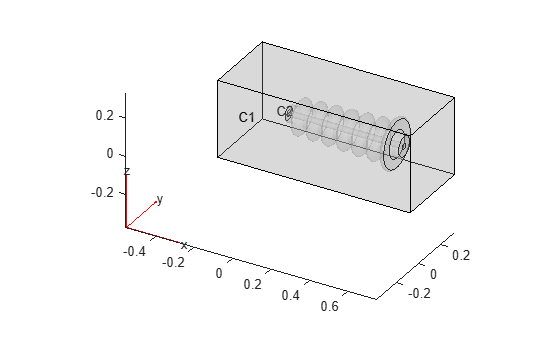Include the geometry in the model.

`model.Geometry = gmModel;`

Specify the vacuum permittivity value in the SI system of units.

`model.VacuumPermittivity = 8.8541878128E-12;`

Specify the relative permittivity of the air.

`electromagneticProperties(model,'Cell',1,'RelativePermittivity',1);`

Specify the relative permittivity of the bushing insulator.

`electromagneticProperties(model,'Cell',2,'RelativePermittivity',5);`

Before specifying boundary conditions, identify the face IDs by plotting the geometry with the face labels. To see the IDs more clearly, rotate the geometry.

```pdegplot(gmModel,'FaceLabels','on','FaceAlpha',0.2) view([55 5])```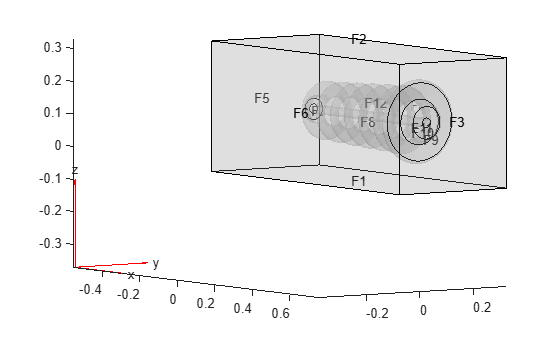Specify the voltage boundary condition on the inner walls of the bushing exposed to conductor.

`electromagneticBC(model,'Face',12,'Voltage',10E3);`

Specify the grounding boundary condition on the surface in contact with the oil tank.

`electromagneticBC(model,'Face',9,'Voltage',0);`

Generate a mesh and solve the model.

```generateMesh(model); R = solve(model)```
```R = ElectrostaticResults with properties: ElectricPotential: [40956x1 double] ElectricField: [1x1 FEStruct] ElectricFluxDensity: [1x1 FEStruct] Mesh: [1x1 FEMesh] ```

Plot the voltage distribution in the bushing.

```elemsBushing = findElements(model.Mesh,'Region','Cell',2); pdeplot3D(model.Mesh.Nodes, ... model.Mesh.Elements(:,elemsBushing), ... 'ColorMapData',R.ElectricPotential);```Plot the magnitude of the electric field intensity in the bushing.

```Emag = sqrt(R.ElectricField.Ex.^2 + ... R.ElectricField.Ey.^2 + ... R.ElectricField.Ez.^2); pdeplot3D(model.Mesh.Nodes, ... model.Mesh.Elements(:,elemsBushing), ... 'ColorMapData',Emag);```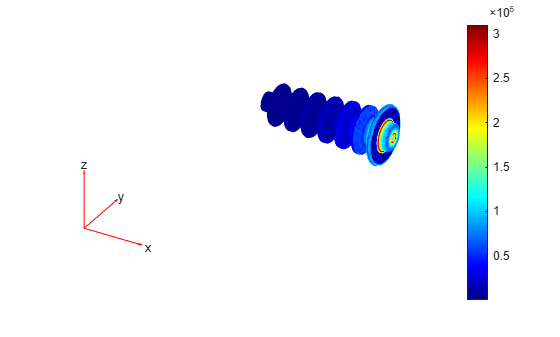## SupportGet trial now